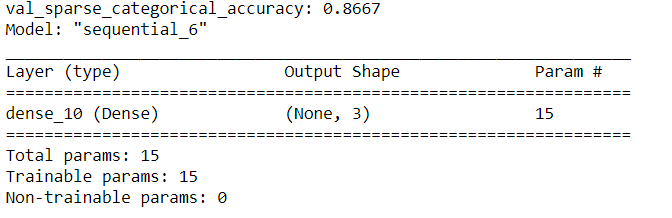model.compile(optimizer='rmsprop', loss=None, metrics=None)

1. optimizer
指定优化器，分别有前面一章中的5种，分别是： sgd、sgdm 、adagrad、rmsprop、adam等优化器 另一种写法为：
tf.keras.optimizers.SGD(learning_rate=0.1)

2. loss
指定损失函数，如mse、categorical_corssentropy、sparse_categorical_corssentropy等
tf.keras.losses.CategoricalCrossentropy(from_logits=False)
tf.keras.losses.MeanSquaredError()
tf.keras.losses.SparseCategoricalCrossentropy(from_logits=False)

from_logits是在询问是否是没有概率分布的输出，所谓的概率分布的输出指的是经过了softmax的效果，经过了概率分布则是False。
3. metrics
网络评测度量，分别有：accuracy、categorical_accuracy、sparse_categorical_accuracy等 accuracy表示y_和y都是以数值形式给出的； categorical_accuracy表示y_和y都是独热编码（概率分布），如：y_=[0,1,0]，y=[0.2, 0.5. 0.3]； sparse_categorical_accuracy表示y_是数值形式，y都是独热编码（概率分布），如：y_=，y=[0.2, 0.5. 0.3]；
import tensorflow as tf
from sklearn import datasets
import numpy as np

np.random.seed(116)
np.random.shuffle(x_train)
np.random.seed(116)
np.random.shuffle(y_train)
tf.random.set_seed(116)

model = tf.keras.models.Sequential([
tf.keras.layers.Dense(3, activation='softmax', kernel_regularizer=tf.keras.regularizers.l2())
])

model.compile(optimizer=tf.keras.optimizers.SGD(lr=0.1),
loss=tf.keras.losses.SparseCategoricalCrossentropy(from_logits=False),
metrics=['sparse_categorical_accuracy'])
迭代打印一次当前的
# 验证集划分为0.2， 每20次sparse_categorical_accuracy
model.fit(x_train, y_train, batch_size=32, epochs=500, validation_split=0.2, validation_freq=20)

model.summary()展开全文tensorflow
• ## tensorflow中model.compile()用法

万次阅读 多人点赞 2020-05-31 21:54:58
tensorflow中model.compile()用法 model.compile()方法用于在配置训练方法时，告知训练时用的优化器、损失函数和准确率评测标准 model.compile(optimizer =优化器， loss =损失函数， metrics = ["准确率”...
tensorflow中model.compile()用法

model.compile()方法用于在配置训练方法时，告知训练时用的优化器、损失函数和准确率评测标准

model.compile(optimizer = 优化器，
loss = 损失函数，
metrics = ["准确率”])
其中：
optimizer可以是字符串形式给出的优化器名字，也可以是函数形式，使用函数形式可以设置学习率、动量和超参数
例如：“sgd”   或者   tf.optimizers.SGD(lr = 学习率，
decay = 学习率衰减率，
momentum = 动量参数）
decay = 学习率衰减率）
decay = 学习率衰减率）
decay = 学习率衰减率）

loss可以是字符串形式给出的损失函数的名字，也可以是函数形式
例如：”mse" 或者 tf.keras.losses.MeanSquaredError()
"sparse_categorical_crossentropy"  或者  tf.keras.losses.SparseCatagoricalCrossentropy(from_logits = False)
损失函数经常需要使用softmax函数来将输出转化为概率分布的形式，在这里from_logits代表是否将输出转为概率分布的形式，为False时表示转换为概率分布，为True时表示不转换，直接输出

Metrics标注网络评价指标
例如：
"accuracy" : y_ 和 y 都是数值，如y_ =  y =   #y_为真实值，y为预测值
“sparse_accuracy":y_和y都是以独热码 和概率分布表示，如y_ = [0, 1, 0], y = [0.256, 0.695, 0.048]
"sparse_categorical_accuracy" :y_是以数值形式给出，y是以 独热码给出，如y_ = , y = [0.256 0.695, 0.048]

示例代码：

#第一步，import
import tensorflow as tf #导入模块
from sklearn import datasets #从sklearn中导入数据集
import numpy as np #导入科学计算模块
import keras

#第二步，train, test

np.random.seed(120) #设置随机种子，让每次结果都一样，方便对照

np.random.shuffle(x_train) #使用shuffle()方法，让输入x_train乱序

np.random.seed(120) #设置随机种子，让每次结果都一样，方便对照

np.random.shuffle(y_train) #使用shuffle()方法，让输入y_train乱序

tf.random.set_seed(120) #让tensorflow中的种子数设置为120

#第三步，models.Sequential()
model = tf.keras.models.Sequential([ #使用models.Sequential()来搭建神经网络
tf.keras.layers.Dense(3, activation = "softmax", kernel_regularizer = tf.keras.regularizers.l2()) #全连接层，三个神经元，激活函数为softmax,使用l2正则化
])

#第四步，model.compile()
model.compile(  #使用model.compile()方法来配置训练方法
optimizer = tf.keras.optimizers.SGD(lr = 0.1), #使用SGD优化器，学习率为0.1
loss = tf.keras.losses.SparseCategoricalCrossentropy(from_logits = False), #配置损失函数
metrics = ['sparse_categorical_accuracy'] #标注网络评价指标
)

#第五步，model.fit()
model.fit(  #使用model.fit()方法来执行训练过程，
x_train, y_train, #告知训练集的输入以及标签，
batch_size = 32, #每一批batch的大小为32，
epochs = 500, #迭代次数epochs为500
validation_split = 0.2, #从测试集中划分80%给训练集
validation_freq = 20 #测试的间隔次数为20
)

#第六步，model.summary()
model.summary() #打印神经网络结构，统计参数数目


展开全文人工智能 深度学习 tensorflow 机器学习
• 主要介绍了keras:model.compile损失函数的用法，具有很好的参考价值，希望对大家有所帮助。一起跟随小编过来看看吧
• 在TensorFLow2中进行神经网络模型的训练主要包括以下几个主要的步骤： 导入相关模块import 准备数据，拆分训练集train、测试集test ...model.compile()的作用就是为搭建好的神经网络模型设置损失函数loss、优化器opt
在TensorFLow2中进行神经网络模型的训练主要包括以下几个主要的步骤：
导入相关模块import准备数据，拆分训练集train、测试集test搭建神经网络模型model (两种方法：Sequential或自定义模型class)模型编译model.compile()模型训练model.fit()查看模型model.summary()模型评价模型预测model.predict()
model.compile()的作用就是为搭建好的神经网络模型设置损失函数loss、优化器optimizer、准确性评价函数metrics。
这些方法的作用分别是：
损失函数和优化器用在反向传播的时候，我们会求损失函数对训练变量的导数，即梯度，然后根据选择的优化器来确定参数更新公式，根据公式对可训练参数进行更新。准确性评价函数用在评估模型预测的准确性。在模型训练的过程中，我们会记录模型在训练集、验证集上的预测准确性，之后会据此绘制准确率随着训练次数的变化曲线。通过查看和对比训练集、测试集随着训练次数的准确率曲线，我们能发现模型是否是过拟合、欠拟合，或者也可以发现多少轮后可以停止模型训练了。
由上可以看出，神经网络模型建模训练的过程中，核心的灵魂环节就是搭建模型和编译compile了。所以，这是非常非常重要的一个模块。
1、首先，上代码，直观看下model.compile()在神经网络建模中的使用示例
#model.compile()配置模型训练方法
model.compile(
optimizer = tf.keras.optimizers.SGD(lr = 0.1), #使用SGD优化器，学习率为0.1
loss = tf.keras.losses.SparseCategoricalCrossentropy(from_logits = False), #配置损失函数
metrics = ['sparse_categorical_accuracy'] #标注准确性评价指标
)
2、解读model.compile()中配置方法
compile(optimizer,  #优化器
loss=None,  #损失函数
metrics=None,   # ["准确率”]
loss_weights=None,
sample_weight_mode=None,
weighted_metrics=None,
target_tensors=None)
2.1  loss可以是字符串形式给出的损失函数的名字，也可以是函数形式

例如：”mse" 或者 tf.keras.losses.MeanSquaredError()
"sparse_categorical_crossentropy"  或者  tf.keras.losses.SparseCatagoricalCrossentropy(from_logits = False)

损失函数经常需要使用softmax函数来将输出转化为概率分布的形式，在这里from_logits代表是否将输出转为概率分布的形式，为False时表示转换为概率分布，为True时表示不转换，直接输出
2.2  optimizer可以是字符串形式给出的优化器名字，也可以是函数形式，使用函数形式可以设置学习率、动量和超参数

例如：“sgd”   或者   tf.optimizers.SGD(lr = 学习率，
decay = 学习率衰减率，
momentum = 动量参数）
decay = 学习率衰减率）
decay = 学习率衰减率）
decay = 学习率衰减率）

2.3 Metrics神经网络模型的准确性评价指标
例如：

"accuracy" : y_ 和 y 都是数值，如y_ =  y =   #y_为真实值，y为预测值
“sparse_accuracy":y_和y都是以独热码 和概率分布表示，如y_ = [0, 1, 0], y = [0.256, 0.695, 0.048]
"sparse_categorical_accuracy" :y_是以数值形式给出，y是以 独热码给出，如y_ = , y = [0.256 0.695, 0.048]


展开全文• 如何为"keras.model.compile"的loss指定输入呀深度学习 keras
• ## Tensorflow2.0 model.compile详细介绍

千次阅读 多人点赞 2020-04-29 16:17:10
Tensorflow2.0 model.compile详细介绍 语法结构：model.compile(loss=‘目标函数’, optimizer=optimizer, metrics=[‘accuracy’]) 1.目标函数，也叫损失函数，是网络中的性能函数，它是一个模型必备的两个参数之一...tensorflow 深度学习
• ## model.compile()函数

千次阅读 2020-08-15 11:14:47python tensorflow
• model.compile(loss= , optimizer= ,metrics= ) 1.loss= "="等于号后面可以加上函数，也可以使用函数代号，两种方式都可以，注意，如果是自定义的损失函数，需要特殊处理才能使用代号，否则只能使用具体函数名称。 ...tensorflow
• poisson：即(predictions - targets * log(predictions))的均值 cosine_proximity：即预测值与真实标签的余弦距离平均值的相反数 补充知识：keras.model.compile() 自定义损失函数注意点 基本用法 model.compile...
• 关于model.compile方法中metrics评价函数的总结 问题引入 　大家会发现我们在做实验的过程中，经常会发现在Model.compile的过程中会需要写一个参数比如：metrics=['accuracy']，那么这个时候一般情况下很少有文章...深度学习 算法 人工智能 python
• 概述 损失函数是模型优化的目标，所以又叫目标函数、优化评分函数，在...model.compile(loss='mean_squared_error', optimizer='sgd') 或者 from keras import losses model.compile(loss=losses.mean_squared_err...
• ## Keras，model.compile详解

万次阅读 多人点赞 2019-06-21 17:13:27
keras model.compile(loss='目标函数 ', optimizer='adam', metrics=['accuracy']) 深度学习笔记 目标函数的总结与整理 　目标函数，或称损失函数，是网络中的性能函数，也是编译一个模型必须的两个参数之一。...
• model.compile( optimizer, loss = None, metrics = None, loss_weights = None, sample_weight_mode = None, weighted_metrics = None, target_tensors = None ) optimizer：优化器，用于控制梯度...keras python 深度学习
• Model.compile( optimizer="rmsprop", loss=None, metrics=None, loss_weights=None, weighted_metrics=None, run_eagerly=None, steps_per_execution=None, **k.
• model.compile(optimizer, loss=None, metrics=None, loss_weights=None, sample_weight_mode=None, weighted_metrics=None, target_tensors=None, **kwargs) Configures the model...
• ## keras笔记——model.compile()函数

万次阅读 多人点赞 2019-01-13 10:43:04
• model.compile 通过调用 compile 方法配置该模型的学习流程： optimizer：优化器，如Adam loss：计算损失，这里用的是交叉熵损失 metrics: 列表，包含评估模型在训练和测试时的性能的指标，典型用法是metrics=...
• 使用keras可以方便的构造出我们自己的模型，但是由于...model.compile中有几个主要的参数，其大概如下： model.compile(loss=[None, total, total, total, total, total, total], loss_weights=[0, 20, 1, 1, 10, 1python 深度学习
• keras model.compile(loss='目标函数 ', optimizer='adam', metrics=['accuracy']) 深度学习笔记 目标函数的总结与整理 目标函数，或称损失函数，是网络中的性能函数，也是编译一个模型必须的两个参数之一。由于损失...
• model.compile(optimizer=Adam(lr=1e-4), loss='binary_crossentropy', metrics=['accuracy']) 注意 loss后类似’binary_crossentropy’、’mse’等代称 loss为函数名称的时候，不带括号 函数参数必须为(y_true, ...tensorflow keras 深度学习keras 损失函数
• 在利用TensorFlow的TensorBoard对train_on_batch...在讲解train_on_batch之前，先看一下Keras的model.compile函数。下面利用Keras版Faster R-CNN代码进行讲解。示例代码如下： # define the RPN, built on the bas...TensorFlow Keras
• ## keras： model.compile ()

万次阅读 2019-04-17 11:30:42
是keras自带的函数,函数位于keras/engine/training.py,是类Model(Network)的一个成员函数,函数原型 def compile(optimizer, loss=None, metrics=None, loss_weights=None, sample_wei...
• https://tensorflow.google.cn/api_docs/python/tf/keras/Sequential?version=stable#compile ...Configures the model for training， 配置模型，准备训练 定义 def compile(self, optimizer='rmsprop', ...
• keras model.compile(loss='目标函数 ', optimizer=‘adam’, metrics=[‘accuracy’])中loss函数详解 原文链接：https://www.cnblogs.com/smuxiaolei/p/8662177.html 深度学习笔记 目标函数的总结与整理   ...
• 损失函数loss：该参数为模型试图最小化的目标函数，它可为预定义的损失函数名，如categorical_crossentropy、mse，也可以为一个损失函数。详情见losses 可用的损失目标函数： mean_squared_error或mse ......